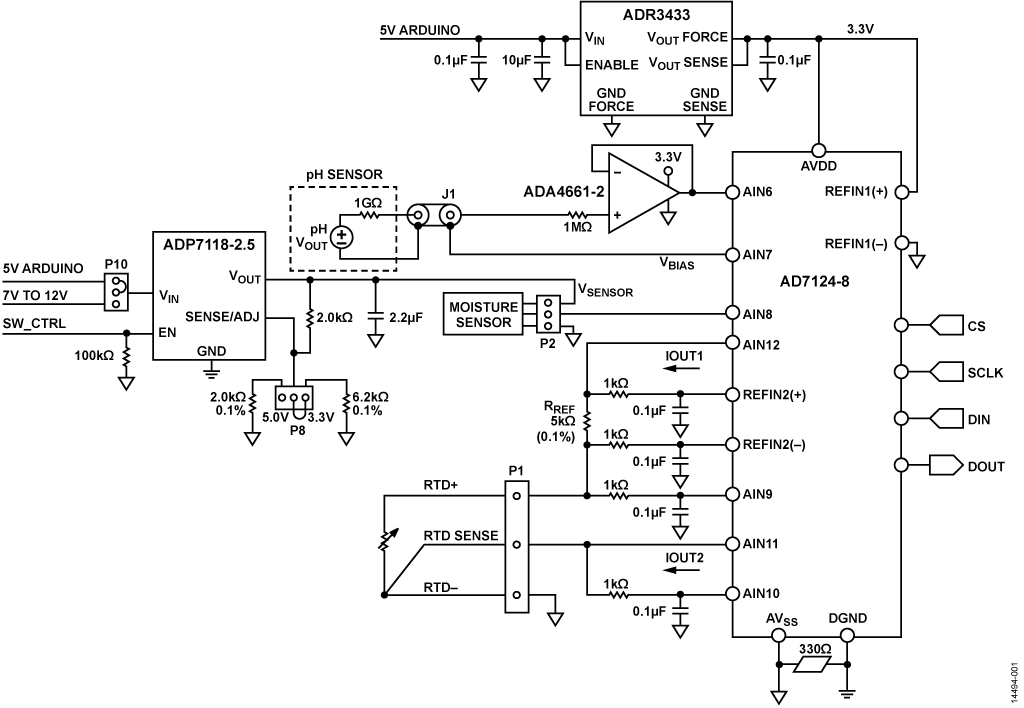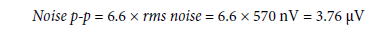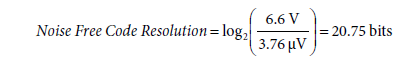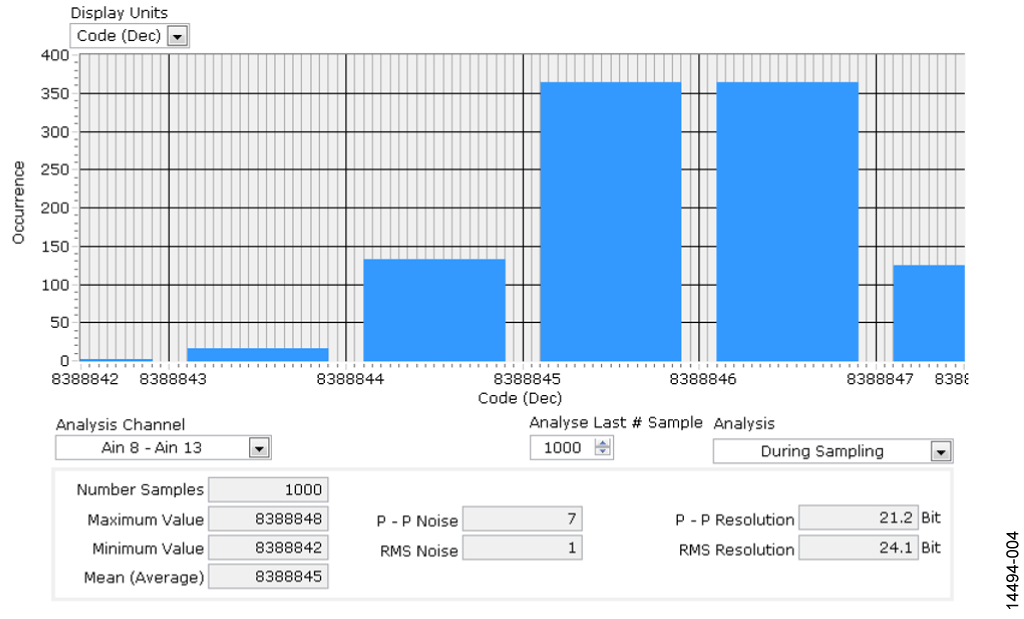Evaluation Hardware \$70.00Overview

Design Resources

Design & Integration Files

• Schematic
• Bill of Materials
• Gerber Files
• Layout Files (Allegro)
• Assembly Drawing Download Design Files 1.04 M

Evaluation Hardware

Part Numbers with "Z" indicate RoHS Compliance. Boards checked are needed to evaluate this circuit

• EVAL-CN0398-ARDZ (\$70.00) EVAL-CN0398-ARDZ

Device Drivers

Software such as C code and/or FPGA code, used to communicate with component's digital interface.

Features & Benefits

• Complete Soil Moisture, pH, and Temperature Measurement
• Low Power
• Arduino-Compatible Form Factor

Circuit Function & Benefits

The circuit shown in Figure 1 is a single-supply, low power, high precision complete solution for soil moisture and pH measurements, including temperature compensation. The circuit is optimized for use with capacitive soil moisture sensors that are insensitive to water salinity and do not corrode over time.

The circuit also measures soil pH and that function is suitable in a variety of applications.

The total current required by the circuit excluding the moisture sensor is only 1.95 mA maximum. The additional power required for the moisture sensor can be minimized by enabling it only for short periods of time using a pulse width modulated (PWM) signal.

The printed circuit board (PCB) is designed in an Arduino-compatible shield form factor and interfaces to the EVAL-ADICUP360 Arduino-compatible platform board for rapid prototyping.Figure 1. Simplified Circuit Block Diagram

Circuit Description

The system is divided into three independent measurement front ends: pH, soil moisture, and temperature. After signal conditioning, the three channels share an AD7124-8 24-bit, Σ-Δ, analog-to-digital converter (ADC). The AD7124-8 is a low power, low noise, completely integrated analog front end for high precision measurement applications. The device contains a low noise, 24-bit, Σ-Δ ADC, and can be configured to have 8 differential inputs or 15 single-ended or pseudo differential inputs. The on-chip gain stage ensures that signals of small amplitude can be interfaced directly to the ADC.

When the ADC is configured for bipolar code operation, the output code for any analog input voltage can be represented aswhere:
N = 24.
Gain is the gain setting (1 to 128).
VREF is the external reference voltage connected between REFIN(+) and REFIN(−) of the AD7124-8.

The AD7124-8 uses the ADR3433, a low cost, low power, high precision CMOS voltage reference, featuring ±0.1% initial accuracy, low operating current, and low output noise. The ADR3433 provides both the 3.3 V reference and also the AVDD supply to the AD7124-8. The ADR3433 can source current up to 10 mA.

Soil pH Measurement

The circuit uses the ADA4661-2 precision op amp to buffer the high impedance pH probe output and to drive the ADC. The ADA4661-2 is a dual, precision, rail-to-rail, input/output amplifier optimized for low power, high bandwidth, and wide operating supply voltage range. Typical input bias current is 0.15 pA to minimize offset errors caused by the bias current flowing through the high output impedance (approximately 1 GΩ) of the pH sensor. The offset voltage of the ADA4661-2 is only 150 μV.

Soil properties are determined by a number of parameters. Soil texture is determined by the mineral particles that include sand, silt and clay. Soil also contains organic matter (living and dead), air, and water. Dissolved chemicals cause the water in the soil to be acidic or alkaline. Soil acidity and alkalinity are measured in units of pH. The pH scale is from 0 (most acid) to 14 (most alkaline), and a pH of 7 is neutral.

A soil pH of 5.2 to 8.0 provides optimum conditions for most agricultural plants. All plants are affected by the extremes of pH, but there is wide variation in their tolerance to acidity and alkalinity. Some plants grow well over a wide pH range, while others are very sensitive to small variations in acidity or alkalinity.

The combination pH electrode can be used for pH measurements. It consists of a glass electrode concentrically surrounded by the reference electrode. The pH electrode generates a small dc voltage corresponding to the soil pH. The measured pH is evaluated to determine if it is within the desired pH range for the specific crop to be grown. Method are then used to correct soil acidity by raising the pH (adding an acid neutralizer such as calcium), or by reducing alkalinity by lowering the pH (adding sulfur, for example).

The output of the pH sensor is bipolar and gives a maximum signal of ±414 mV at 25°C. The AD7124-8 operates from a single power supply therefore the pH probe should be biased above ground so that it is within the acceptable common-mode range of the AD7124-8. One of the integrated features of the AD7124-8 is its internal bias voltage generator that sets the common-mode voltage of a channel to AVDD/2, or 1.65 V. This bias voltage from the ADC is applied to the pH probe shield and sets the output of the sensor 1.65 V ±414 mV at 25°C.

Two-Point pH Calibration

The characteristics of pH electrode change with time due to electrode coating and aging, therefore a calibration procedure is required to obtain maximum accuracy.

Calibration is performed by measuring the pH of two buffer solutions, each with a known pH. The software includes NIST lookup tables for different pH buffer solutions and includes the pH temperature corrected pH values from 0°C to 95°C. The RTD is used to measure the temperature of the solution.

Using the following linear equation,The actual slope of the pH sensor transfer function is determined, and the actual offset voltage measured. To calculate the slope, solve the following equation form:where:
y1 is the measured voltage at first point.
y2 is the measured voltage at second point.
x1 is the known pH at first point.
x2 is the known pH at second point.

After taking the above measurements and substituting one of the calibration points into Equation 2, getting the unknown pH can be determined from the following final equation:where:
x is the unknown soil pH.
y is the measured voltage.
b is the measured offset voltage.
m is the slope.

For direct measurement where no calibration is performed, unknown soil pH can be determined by solving the following Nernst Equation for pH:where:
E is the measured electrode voltage of the unknown soil pH.
α is the measured offset voltage.
T is the measured temperature in °C.
n = 1 at 25oC, valence (number of charges on ion) .
F = 96,485 coulombs/mol, Faraday constant.
R = 8.314 volt-coulombs /K mol, Avogadro’s number.
pH is the hydrogen ion concentration of an unknown solution.
pHISO = 7, reference hydrogen ion concentration.

Soil Moisture Measurement

An important factor affecting crop growth and yield is the soil water content. Soil moisture measurement is therefore key to water conservation in agriculture irrigation systems. Most of the common soil moisture sensors used in the industry today are capacitive type sensors. A capacitive sensor measures the water content of the soil, where the volume of water influences the dielectric constant within the total volume of the soil. Water has a relative dielectric constant, εr = 80, which is much greater than the relative dielectric constant of other elements in the soil, such as mineral soil (εr = 4), organic matter (εr = 4), and air (εr = 1). Changes in the amount of water content in the soil therefore correspond to capacitance changes due to changes in dielectric constant.

The moisture sensor converts the measured capacitance between the probes into a dc voltage that can interface directly to the ADC for conversion into corresponding volumetric water content (VWC).

The CN-0398 moisture sensor circuit accepts the output of a 3-wire moisture sensor (power, ground, and voltage output) with an output voltage range from 0 V to 3 V. A moisture sensor, such as Decagon Devices EC-5, requires PWM excitation pulses that leave sensors turned off most of the time. The ADP7118-2.5 LDO enable pin (SW_CTRL label) is used to turn the power to the sensor on an off with a PWM pulse.

The ADP7118-2.5 is a CMOS, low dropout (LDO) linear regulator that operates from 2.7 V to 20 V and provides up to 200 mA of output current. The input to the ADP7118-2.5 is selectable via the jumper P10 as either 5 V or 7 V to 12 V coming from the Arduino- compatible platform board. The output of the ADP7118-2.5 is also selectable as 3.3 V or 5 V using the jumper on P8.

When turning the sensor power on and off with a PWM signal, the stabilization times of the sensors must be observed before sampling the output. The Decagon EC-5 sensor requires 10 ms, and the Vegetronix VH400 requires 400 ms.

Voltage to VWC Conversion
The circuit was evaluated using the Decagon Devices EC-5 moisture sensor that is factory calibrated and has the following conversion function:where:
VWC is the volumetric water content of the soil in %.
mV is the sensor output in mV.

The conversion function for the Vegetronix VH400 moisture sensor is a piecewise linear approximation shown in Table 1.

 Voltage Range Equation (V = Sensor Output in Volts) 0 V to 1.1 V VWC = 10 × V − 1 1.1 V to 1.3 V VWC = 25 × V − 17.5 1.3 V to 1.82 V VWC = 48.08 × V − 47.5 1.82 V to 2.2 V VWC = 26.32 × V − 7.89

Temperature Measurement

The temperature measurement circuit in Figure 2 is a 3-wire Pt100 resistance temperature detector (RTD) system based on the AD7124-8 24-bit, Σ-Δ ADC. The pH measurement can be compensated for temperature effects using the RTD temperature. The AD7124-8 has a programmable low drift excitation current sources from 50 μA to 1 mA. The ADC channels AIN11 and AIN12 are programmed for excitation currents of 500 μA. IOUT1 (AIN11) flows through the 5.11 kΩ reference resistor and the RTD. Since the same current flows through the RTD and the reference resistor, the measurement is ratiometric, and any errors due to variations in the excitation current are removed. IOUT2 (AIN12) flows into the RTD RL2 lead resistance and generates a voltage that cancels the voltage dropped across the RL1 lead resistance.Figure 2. RTD-Based Temperature Measurement Circuit

Temperature compensation is usually needed in a pH because temperature greatly affects the sensitivity of the pH probe. The pH probe ideally produces 59.154 mV/pH at 25°C, but varies depending on the actual temperature of the sample being measured.

The 4-wire RTD used for the cold junction requires its own linearization. The general expression to calculate the RTD resistance (R) where the ADC is operating in bipolar mode is given bywhere:
RRTD is the resistance of the RTD.
CODE is the ADC code.
N is the resolution of the ADC, 24.
RREF is the reference resistor.
G is the selected gain, 16.

The steps involved in converting the RTD resistance to a temperature and the linearization process are outlined in the Circuit Note CN-0381.

Predicting System Noise Performance of pH Channel

For an output data rate of 25 SPS and a gain of 1, the rms noise of the AD7124-8 is 570 nV in the full power mode (noise is referred to input, taken from AD7124-8 data sheet). The peak- to-peak noise is thereforeUsing rss to add the noise contributed by the ADA4661-2 (3 μV p-p), the total predicted system noise is 4.818 μV p-p. If the pH meter has a sensitivity of 59 mV/pH, the pH meter therefore measures the pH level to a noise-free resolution ofThe full-scale ADC input range is 6.6 V. Therefore, the predicted peak-to-peak resolution isPredicting System Noise Performance of Moisture Channel and Temperature Channel

The moisture sensor and the RTD connect directly to the ADC input, therefore the shorted input noise free code resolution is primarily determined by the AD7124-8 noise which is 570 nV rms that is equivalent to 3.76 uV p-p. The noise free code resolution is then calculated:Actual System Noise Performance

For the pH measurement, the actual system peak-to-peak noise and resolution was determined by shorting the input pH probe BNC connector and acquiring 1000 samples. As shown in the Figure 3 histogram, system peak-to-peak resolution is 18.2 bits compared to the predicted value of 20.38 bits. Measurements were done at full power mode, 25 SPS, and the post filter was used.Figure 3. Shorted Input Histogram for pH Channel

For the moisture measurement, the actual system peak-to-peak noise and resolution was determined by shorting the input of the moisture sensor connector and acquiring 1000 samples. As shown in the Figure 4 histogram, system noise free code resolution is 21.2 bits. Measurements were done at full power mode, 25 SPS, and the post filter was used.Figure 4. Shorted Input Histogram for Moisture Sensor Channel

Soil Moisture Test Repeatability Measurements

Table 2 and Table 3 show the data output of the moisture sensors when probing the same sample of some sandy soil. The Decagon EC-5 and Vegetronix VH400 and moisture sensors were used during the evaluation. The soil was probed seven times with each sensor and the average calculated. The results show a repeatability of better than 1% in the VWC measurement.

 Test Output (mV) VWC (%) 1 544.70 9.03 2 542.10 8.78 3 546.57 9.22 4 537.74 8.34 5 546.74 9.24 6 542.89 8.85 7 552.70 9.83 Average 544.78 9.04

 Test Output (V) VWC (%) 1 1.04 0.39 2 0.93 8.28 3 1.01 9.07 4 1.04 9.42 5 1.03 9.30 6 0.99 8.86 7 1.06 9.63 Average 1.01 9.14

Soil Moisture Content Determination

VWC is defined as the volume of water per volume of bulk soil:where:
θ is volumetric water content (cm3/cm3).
VW is the volume of water (cm3).
VT is the total volume of bulk soil sample (cm3).

The following empirical procedure can be used to determine the VWC of a soil sample independent of electronic measurements. A beaker and scale are required.

1. Obtain a soil sample
2. Weigh the beaker without soil
3. Put a known volume of moist soil into the beaker
4. Weigh the beaker with moist soil (grams)
5. Dry the soil using an oven for 24 hours at 110°C
6. Weigh the dried soil (grams)

The mass of the water removed from the soil iswhere:
mW is the mass of the water in the soil.
mWET is the mass of the moist soil.
mDRY is the mass of the dry soil.

Because water density is 1 g/cm3, the mass of the water is equal to the volume. This value is used in the determination of the VWC.

This procedure is described in complete detail in the Decagon Devices Application Note, Calibrating ECH2O Soil Moisture Sensors.

Table 4 shows the calculation of VWC based on adding known volumes of water to a sample of sandy soil that had previously been dried in an oven.

The Decagon EC-5 and Vegetronix VH400 sensors were used to measure the VWC at each of the five water concentration levels. The factory recommended equations were used to convert the voltage output of each of the sensors to VWC as shown in the tables.

Figure 5 shows the VWC response of the EC-5 moisture sensor (Table 5) compared to the value calculated based on the actual water volume (Table 4). Note that the worst case error is approximately 3%.

 Dried Soil Volume (cm3) Water Volume (cm3) Calculated VWC (%) 187.136 18.672 9.98 187.136 31.531 16.85 187.136 46.789 25.00 187.136 58.8 31.42 187.136 71.089 37.99

 Dried Soil Volume (cm3) Water Volume (cm3) Sensor Output (mV) Output Converted the VWC (%) 187.136 18.672 548 9.3616 187.136 31.531 593 13.8256 187.136 46.789 677 22.1584 187.136 58.8 779 32.2768 187.136 71.089 849 39.2208Figure 5. Actual vs. Measured VWC for Decagon EC-5 Sensor Using Data Sheet Equation

Soil-specific calibration may improve the accuracy of the moisture sensor. Different sensors are typically calibrated with a specific soil, but due to variations in soil bulk density, mineralogy, texture, and salinity, accuracy may vary.

The sensor data sheet generally gives recommended equations for converting the sensor output into VWC. However, the accuracy can sometimes be improved by generating an equation that more accurately fits the VWC data points taken from the actual soil under test. Before developing a soil-specific equation based on actual VWC measurements for a particular sensor, first calculate the errors obtained by using the recommended equations in the sensor data sheet. If the errors are 2% or less over the desired range for the soil selected, then there is no reason to develop a soil-specific equation. If the error is greater than several percentage points, then a soil-specific equation based on a least-squares fit straight line may lower the error. In some cases a polynomial function may be required to obtain the desired accuracy, depending on the transfer function of the specific sensor.

In the case of the Decagon EC-5 sensor, a soil-specific straight line fit shown in Figure 6 using the least squares method gave good results and reduced the maximum error from 3% to 1.8%.Figure 6. Actual vs. Measured VWC for Decagon EC-5 Sensor Using Soil-Specific Best Fit Equation

Table 6 shows the measured VWC using the Vegetronix VH400 moisture sensor. The data in Table 6 and Table 4 is plotted in Figure 7.

 Dried Soil Volume (cm3) Water Volume (cm3) Sensor Output (V) Output Converted to VWC (%) 187.136 18.672 1.046 9.46 187.136 31.531 1.362 16.55 187.136 46.789 1.474 23.36992 187.136 58.8 1.673 32.93784 187.136 71.089 1.73 35.6784Figure 7. Actual vs. Measured VWC for Vegetronix VH400 Sensor Using Data Sheet Equations

Figure 7 shows an error of only 2.3% using the data sheet equations; therefore, no soil-specific equation was required for the soil under test with the VH400 sensor.

A complete set of documentation for the EVAL-CN0398-ARDZ board including schematics, layouts, and bill of materials is available in the CN-0398 Design Support package.

Common Variations

Other suitable ADCs for this circuit are the AD7794 and AD7795. Both devices have the same feature set as the AD7124-8. However, the AD7794 is a 6-channel, 24-bit ADC, while the AD7795 is a 6-channel, 16-bit ADC.

The AD8615 buffer amplifier is available in a 5-lead TSOT package. It is a precision, 20 MHz, CMOS, rail-to-rail input/ output op amp with a typical input bias current of 0.2 pA and a low offset voltage of 80 μV (typical).

The ADR4533 ultralow noise, high accuracy voltage reference is also suitable to provide a 3.3 V reference.

The ADP7112 is a CMOS LDO linear regulator and has the same basic features as the ADP7118 but is available in a WLCSP package.

Circuit Evaluation & Test

This circuit uses the EVAL-CN0398-ARDZ shield circuit board, an optional external power supply, PC with serial port terminal program, and the EVAL-ADICUP360 Arduino-compatible platform board. A user guide for the platform board is available on the product page, at www.analog.com/EVAL-ADICUP360 . A user guide for the EVAL-CN0398-ARDZ board and software is available at www.analog.com/CN0398-UserGuide.

Equipment Required

The following equipment is needed:

• EVAL-CN0398-ARDZ circuit board
• EVAL-ADICUP360 Arduino-compatible platform loaded with CN-0398 firmware
• PC with a USB port and 64-bit Linux distribution OS kernel v4.2.0-amd 64 or later, with the terminal program
• 7 V to 12 V/1 A dc power supply, or equivalent 7 V to 12 V/1 A bench supply (not required unless using a 5V moisture sensor)
• Soil
• Beaker
• Drying oven
• Weighing scale
• VH400 moisture sensor
• EC-5 moisture sensor

Test Setup Functional Block Diagram

Figure 8 shows a functional diagram of the test setup.Figure 8. Test Setup Functional Block Diagram

Setup

Take the following step for circuit evaluation:

1. Plug the EVAL-CN0398-ARDZ shield board into the EVAL-ADICUP360 platform board.
2. Connect the sensors to the EVAL-CN0398-ARDZ board.
3. Following the instructions in the EVAL-ADICUP360 user guide, connect the EVAL-ADICUP360 virtual COM USB port to the PC.
4. If using a 5V moisture sensor, power up the EVAL-ADICUP360 with a 7 V to 12 V/1 A dc power supply. Otherwise, the ADICUP360 is powered directly from the USB port.
5. Set up the terminal software using the 115,200 Hz baud rate and the correct the virtual COM port.
6. If the system does not have the correct baud rate setting, set the default value by pressing the RESET button on the EVAL-ADICUP360 board.
7. Run the software, and the software continuously displays the output of the sensors for pH, temperature, and moisture content.

Details of the operation of the hardware and software can be found in the CN-0398 User Guide at www.analog.com/CN0398-UserGuide. The CN-0398 User Guide also contains details of how the software can be modified to accommodate soil-specific calibration using straight line or polynomial equations.

Figure 9 shows a photograph of the EVAL-CN0398-ARDZ Arduino shield board.

Figure 10 shows a photograph of the sample test setup of soil moisture measurement using the EC-5 moisture sensor.Figure 10. EC-5 Sensor Moisture Measurement on Sandy Soil